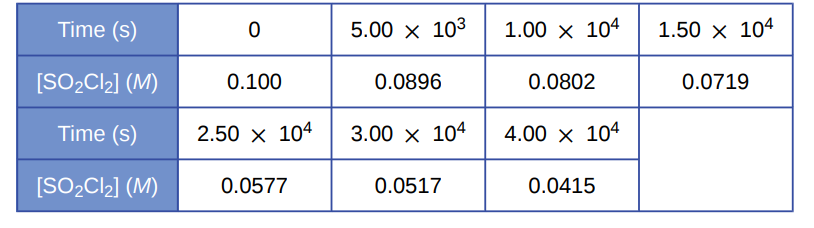# Problem: Use the data provided to graphically determine the order and rate constant of the following reaction: SO2Cl2 ⟶ SO2 + Cl2

###### FREE Expert Solution
81% (124 ratings)
###### Problem Details

Use the data provided to graphically determine the order and rate constant of the following reaction: SO2Cl2 ⟶ SO2 + Cl2Frequently Asked Questions

What scientific concept do you need to know in order to solve this problem?

Our tutors have indicated that to solve this problem you will need to apply the Identifying Reaction Order concept. If you need more Identifying Reaction Order practice, you can also practice Identifying Reaction Order practice problems.

What textbook is this problem found in?

Our data indicates that this problem or a close variation was asked in Chemistry - OpenStax 2015th Edition. You can also practice Chemistry - OpenStax 2015th Edition practice problems.